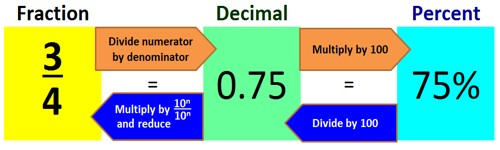Converting Percent to Fraction and Decimal

When we talk, we often use different words to express the same thing. For example, we could describe the same car as tiny or little or small. All of these words mean the car is not big. Fractions, decimals, and percents are like the words tiny, little, and small. They’re all just different ways of expressing parts of a whole.

Converting a Percent to a Fraction

Do the following steps to convert a percent to a fraction:

For example: Convert 83% to a fraction.

• Remove the Percent sign
• Make a fraction with the percent as the numerator and 100 as the denominator (e.g. 83/100)
• Reduce the fraction if needed

Step 1: Write down the percent divided by 100 like this:  percent /100

Step 2: If the percent is not a whole number, then multiply both top and bottom by 10 for every number after the decimal point. (For example, if there is one number after the decimal, then use 10, if there are two then use 100, etc.)

Step 3: Simplify (or reduce) the fractionConverting a Decimal to a Percent

Do the following steps to convert a decimal to a percent:

For example: Convert 0.83 to a percent.

• Multiply the decimal by 100 (e.g. 0.83 * 100 = 83)

Converting a Percent to a Decimal

When we turn a percent into a decimal, we’re actually doing two steps. First, we convert our percent into a fraction. Since all percents are out of 100, we just put the percent over 100, like this:

78% = 78/100

You know that all percents are out of 100, so you can skip making the percent into a fraction. You have to divide the percent by 100 to get a decimal, but there’s a quick way to do that. Just move the decimal point two spaces to the left! This way, you can get the same answer with just one easy step.

How to convert a percent to a decimal:

For example: Convert 83% to a decimal.

• Divide the percent by 100 (e.g. 83 ÷ 100 = 0.83)

Information Source: